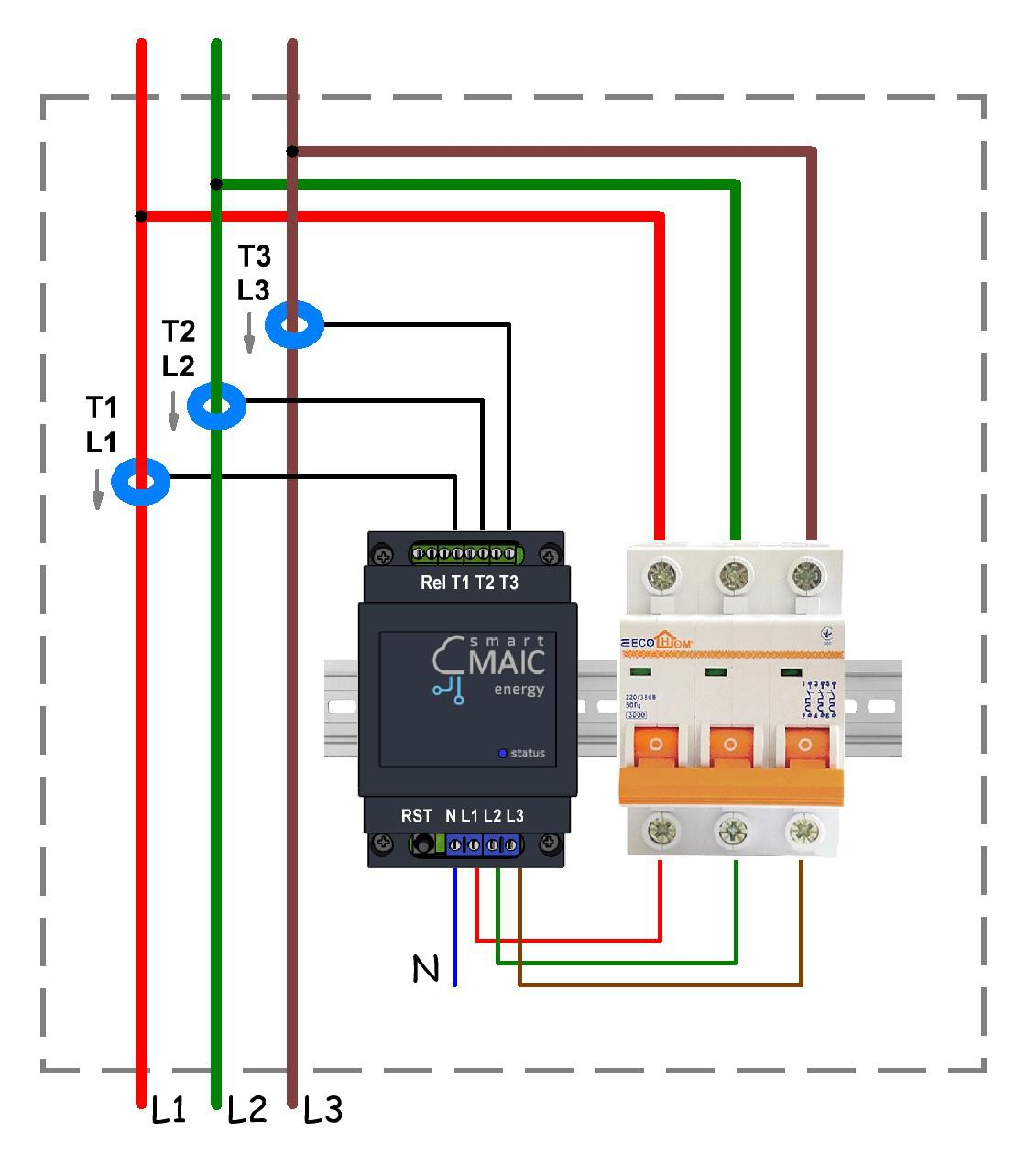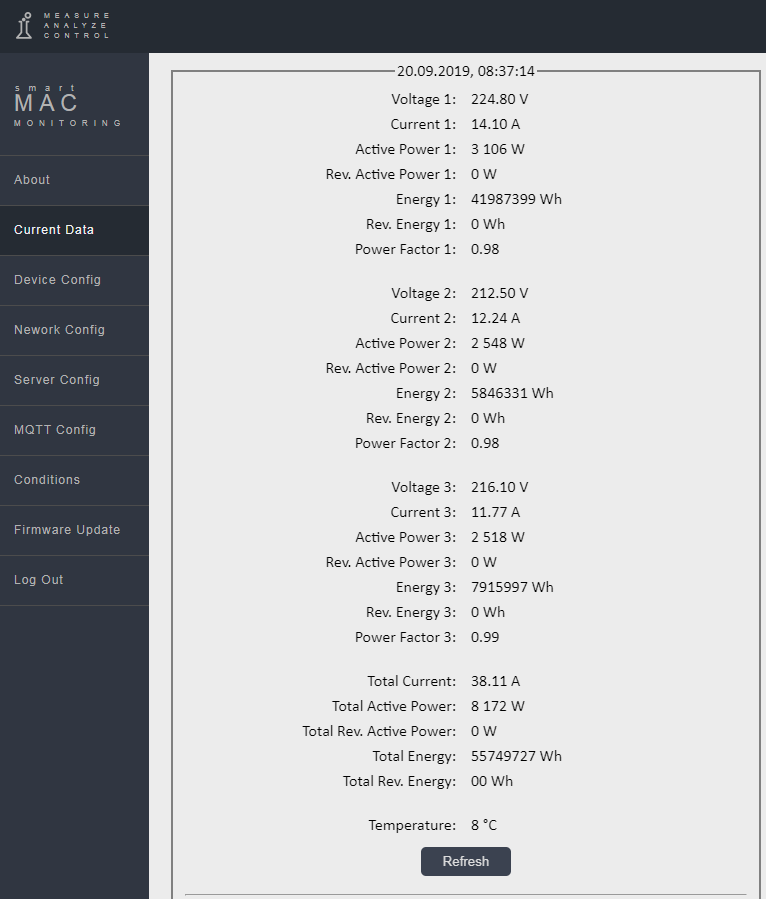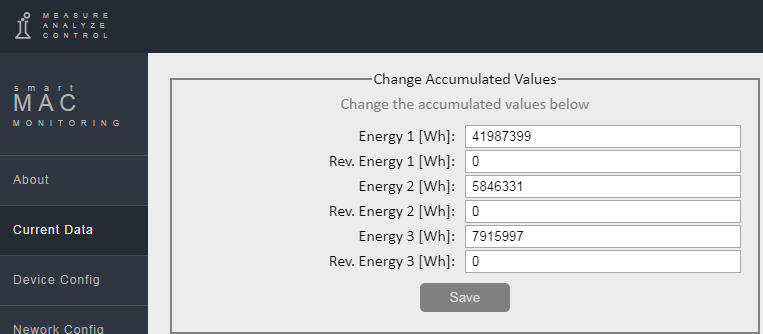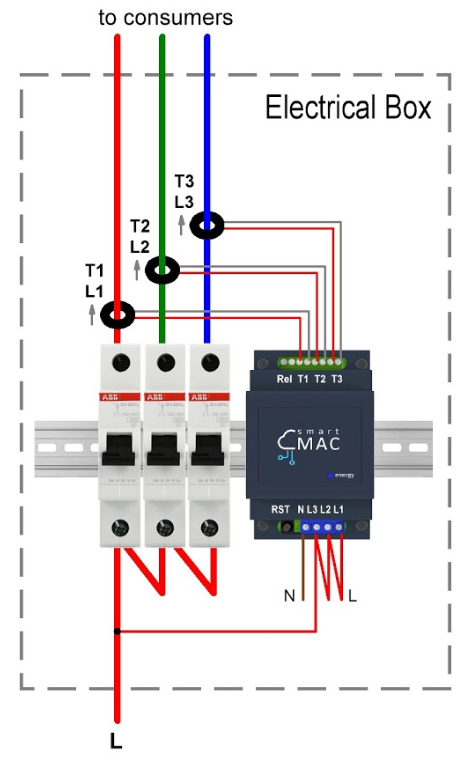# Advanced installation of Energy meter D103

### D103 connection in 3 phase network

Connect current transformers to the device at inputs T1, T2, T3 in accordance with the figure in the installation instructions. Each current transformer is marked with its number and current measurement arrow. Install current transformers on the phase wires by placing the arrow in the direction of the consumer. Center the current transformers so that they are perpendicular to the wires. This orientation provides more accurate measurement results.
Do not install current transformers on two-wire wires through which zeros and phase are connected at the same time, with this connection the measurement result will be 0.
Connect power to inputs N, L1, L2 and L3 of the device. It is important that the power supply matches the line on which the current transformer is installed.After connecting, щзут the device’s Web page in the “Current Data” menu and check the correctness of the readings taken. Check each number, see if the Power Factor [PF] parameter confuses you (usually aim for 1.00) and if reverse appears when it shouldn’t.To synchronize the accumulative counter [Wh] and [rWh] with the readings of your electric meter, set the initial values for the accumulative data in the device settings in the Data section. Please note that data is entered in watts.### Measured data

It is important to understand how the data from the smart-MAC energy monitor is stored at different time intervals. It is especially important to understand the calculation principle for the [W] Power parameter when it is converted from electrical power to electrical energy.

 D103 Energy meter measured data, Designation Real-Time and Minute interval Hourly, Daily, Weekly, Monthly, Annual interval Voltage, V1 V2 V3 Voltage in [V] The arithmetic mean in [V] Current, А1 A2 A3 and TA (total) Current in [A] The arithmetic mean in [A] Power, W1 W2 W3 и TW (total) Instant Active Power [W] Active Energy for the period [Wh] Reverse Power, rW1 rW2 rW3 and TrW (total) Instant reverse Active Power [W] Reverse Active Energy for the period [Wh] Power Factor, PF1  PF2 PF3 Power Factor (cosФ) The arithmetic mean Energy, Wh1 Wh2 Wh3 и TWh (total) Cumulative electricity meter for Active Energy [Wh] The same, cumulative electricity meter [Wh] Reverse Energy, rWh1 rWh2 rWh3 and TrWh (total) Cumulative electricity meter for reverse Active Energy [rWh] The same, cumulative electricity meter [rWh]

As already mentioned, there is an arrow on each current transformer. If the arrow is placed towards the consumer, then the monitoring data will be saved as follows:

- active power (consumption) in the [W] Power;

- reverse active power (generation) in the [rW] Revers Power;
- the accumulative meter of consumed energy in [Wh] Energy;
- accumulative counter of reverse energy in [rWh] Energy.

### D103 single-phase connection

The D103 energy monitor is essentially 3-line. Therefore, it can be installed in a single-phase power for monitoring lines to different consumers (boiler, air conditioning, kitchen, sockets, lighting, etc.)

The circuit is simple. Connect power to one of the inputs and set jumpers for the remaining power inputs l1 l2 L3.
Connect current transformers and put on the appropriate lines that go to consumers.### Reactive Power and Linear Voltage.

For monitoring Reactive Power and Reactive Energy, as well as for monitoring Line Voltage, you can use the calculated values on the widget settings.
See the corresponding article in the Knowledge Base for more details. View »